# Crack SBI Clerk / Syndicate PO / Canara Bank PO 2018 – Logical Reasoning (Miscellaneous) Day-5

## Crack SBI Clerk / Syndicate PO / Canara Bank PO 2018 – Logical Reasoning (Miscellaneous) Day-5:

Dear Readers, Reasoning Section play a vital role in Banking and all other competitive exams. This year 2018 was started with three wonderful opportunities; Syndicate Bank PO Exam, Canara Bank PO Exam and Most Awaited SBI Clerk 2018. To enrich your preparation here we have providing new series of Practice Questions on Reasoning Ability – Miscellaneous. Candidates those who are going to appear in Bank Exams 2018 can practice these questions daily and make your preparation effective.

[WpProQuiz 1236]

### Click “Start Quiz” to attend these Questions and view Solutions

1) How many such pairs of letters are there in the word STARVATION each of which has as many letters between them in the word as in the English alphabet? (In both forward and backward directions)

1. None
2. One
3. Two
4. Three
5. More than three.

2) In a certain code language TREAT is written as UBFSU and HABIT is written as UJCBI.

How is AGREE written in that code language?

1. FSHBF
2. FSHFB
3. FFSHB
4. FFQBH
5. None of these

3) If the letters of the word AMERICA are arranged in the English alphabetical order from left to right, the position of how many letters will remain unchanged?

1. None
2. One
3. Two
4. Three
5. None of these

Directions (4 – 5) Study the following information carefully and answer the questions that follow:

A ÷ B means A is son of B

A × B means A is sister of B

A + B means A is brother of B

A – B means A is mother of B

4) How is G related to H in the expression “G × R + V ÷ H”?

1. Sister
2. Daughter
3. Son
4. Mother
5. None of these

5) Which of the following expressions represents “J is wife of E‟?

1. E ÷ F × G + H – J
2. E × G ÷ H + F – J
3. J – H × G ÷ E + F
4. Both (a) and (b)
5. None of these

Directions (6-10) Study the sets of numbers given below and answer the questions, given below:

489   541    654    953    783

6) If in each number, all the three digits are arranged in ascending order, which of the following will be the lowest number?

1. 489
2. 541
3. 654
4. 953
5. 783

7) If five is subtracted from each of the numbers, which of the following numbers will be the difference between the second digit of second highest number and the second digit of the highest number?

1. Zero
2. 3
3. 1
4. 4
5. 2

8) If in each number the first and the second digits are interchanged, which will be the third highest number?

1. 489
2. 541
3. 654
4. 953
5. 783

9) Which of the following numbers will be obtained if the first digit of lowest number is subtracted from the second digit of highest number after adding one to each of the numbers?

1. 1
2. 2
3. 3
4. 4
5. 5

10)  If in each number, the first and the last digits are interchanged which of the following will be the second highest number?

1. 489
2. 541
3. 654
4. 953
5. 783

1. Answer e) More than three.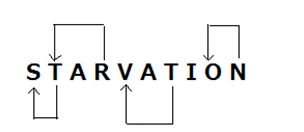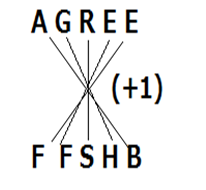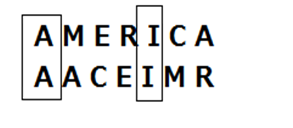4.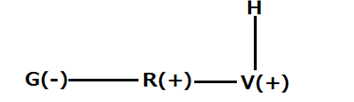5.

Answer c) J – H × G ÷ E + F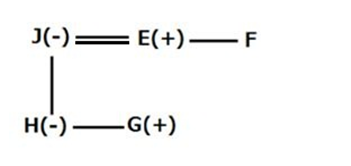Direction (6-10):

489   541    654    953    783

484   145    456    359    378

489   541    654    953    783

489   536    649    948    778

Difference 7 – 4 = 3

489   541    654    953    783

849   451    564    593    873

489   541    654    953    783

490   542    655    954    784

Difference 5 – 4 = 1